# 03 Network Architectures¶

In this notebook, we investigate how we can work with more complicated network architectures than the simple MLP's.

The example will be a Logistic-Hazard which use the encoded (latent) variables of an autoencoder as covariates. This means the network will have two heads, one for the autoencoder and the other for the Logistic-Hazard.

To approach this task we can use the LogisticHazard, but we need to define the network structure that combines the survival net with an autoencoder. Also, we need to define a loss function that combines the loss of the LogisticHazard with that of the autoencoder.

In :
import numpy as np
import matplotlib.pyplot as plt

# For preprocessing
from sklearn.preprocessing import StandardScaler
from sklearn_pandas import DataFrameMapper

import torch # For building the networks
from torch import nn
import torch.nn.functional as F
import torchtuples as tt # Some useful functions

from pycox.datasets import metabric
from pycox.models import LogisticHazard
from pycox.models.loss import NLLLogistiHazardLoss
from pycox.evaluation import EvalSurv

In :
## Uncomment to install sklearn-pandas
# ! pip install sklearn-pandas

In :
np.random.seed(1234)
_ = torch.manual_seed(123)


## Dataset¶

We load the METABRIC data set as a pandas DataFrame and split the data in in train, test and validation.

The duration column gives the observed times and the event column contains indicators of whether the observation is an event (1) or a censored observation (0).

In :
df_train = metabric.read_df()
df_test = df_train.sample(frac=0.2)
df_train = df_train.drop(df_test.index)
df_val = df_train.sample(frac=0.2)
df_train = df_train.drop(df_val.index)

In :
df_train.head()

Out:
x0 x1 x2 x3 x4 x5 x6 x7 x8 duration event
0 5.603834 7.811392 10.797988 5.967607 1.0 1.0 0.0 1.0 56.840000 99.333336 0
1 5.284882 9.581043 10.204620 5.664970 1.0 0.0 0.0 1.0 85.940002 95.733330 1
3 6.654017 5.341846 8.646379 5.655888 0.0 0.0 0.0 0.0 66.910004 239.300003 0
4 5.456747 5.339741 10.555724 6.008429 1.0 0.0 0.0 1.0 67.849998 56.933334 1
5 5.425826 6.331182 10.455145 5.749053 1.0 1.0 0.0 1.0 70.519997 123.533333 0

## Feature transforms¶

The METABRIC dataset has 9 covariates: x0, ..., x8. We will standardize the 5 numerical covariates, and leave the binary covariates as is. Note that PyTorch require variables of type 'float32'.

We like using the sklearn_pandas.DataFrameMapper to make feature mappers.

In :
cols_standardize = ['x0', 'x1', 'x2', 'x3', 'x8']
cols_leave = ['x4', 'x5', 'x6', 'x7']

standardize = [([col], StandardScaler()) for col in cols_standardize]
leave = [(col, None) for col in cols_leave]

x_mapper = DataFrameMapper(standardize + leave)

In :
x_train = x_mapper.fit_transform(df_train).astype('float32')
x_val = x_mapper.transform(df_val).astype('float32')
x_test = x_mapper.transform(df_test).astype('float32')


## Label transforms¶

The LogisticHazard is a discrete-time method, meaning it requires discretization of the event times to be applied to continuous-time data. We let num_durations define the size of this (equidistant) discretization grid, meaning our network will have num_durations output nodes.

Note that we have two sets of targets. The first, y_train_surv, is for the survival and contains the labels (idx_durations, events). The second is for the autoencoder (which is just the input covariates x_train). This is important to note, as it will define the call arguments of our loss function.

In :
num_durations = 10
labtrans = LogisticHazard.label_transform(num_durations)
get_target = lambda df: (df['duration'].values, df['event'].values)
y_train_surv = labtrans.fit_transform(*get_target(df_train))
y_val_surv = labtrans.transform(*get_target(df_val))

train = tt.tuplefy(x_train, (y_train_surv, x_train))
val = tt.tuplefy(x_val, (y_val_surv, x_val))

# We don't need to transform the test labels
durations_test, events_test = get_target(df_test)

In :
y_train_surv

Out:
(array([2, 3, 6, ..., 1, 5, 3]),
array([0., 1., 0., ..., 1., 0., 0.], dtype=float32))

We see that we have combined idx_durations and events intro the tuple y_train_surv.

## The Neural Net¶

We first define our network NetAESurv with contains the encoder, decoder, and the survival part. We also include a predict method that saves computations by not computing the decoder.

In :
class NetAESurv(nn.Module):
def __init__(self, in_features, encoded_features, out_features):
super().__init__()
self.encoder = nn.Sequential(
nn.Linear(in_features, 32), nn.ReLU(),
nn.Linear(32, 16), nn.ReLU(),
nn.Linear(16, encoded_features),
)
self.decoder = nn.Sequential(
nn.Linear(encoded_features, 16), nn.ReLU(),
nn.Linear(16, 32), nn.ReLU(),
nn.Linear(32, in_features),
)
self.surv_net = nn.Sequential(
nn.Linear(encoded_features, 16), nn.ReLU(),
nn.Linear(16, 16), nn.ReLU(),
nn.Linear(16, out_features),
)

def forward(self, input):
encoded = self.encoder(input)
decoded = self.decoder(encoded)
phi = self.surv_net(encoded)
return phi, decoded

def predict(self, input):
# Will be used by model.predict later.
# As this only has the survival output,
# we don't have to change LogisticHazard.
encoded = self.encoder(input)
return self.surv_net(encoded)


All methods in pycox are built on torchtuples.Model. The way torchtuples is made, a call to model.predict will use model.net.predict if it is defined, and use model.net.forward if predict is not defined. As all the survival predictions in pycox are based on the model.predict method, a call to model.predict_surv will use the NetAESurv.predict method instead of the NetAESurv.forward method. This way, the model.predict_surv methods will work as before!

In :
in_features = x_train.shape
encoded_features = 4
out_features = labtrans.out_features
net = NetAESurv(in_features, encoded_features, out_features)


## The Loss¶

We need to define a loss that combines the LogisticHazard loss with the loss of an autoencoder MLELoss. The forward method defines how the loss is called, and need to be defined in accordance with how the data is structured.

The first arguments need to be the output of the net (phi and decoded above), and the remainder of the arguments need to have the same structure as the tuple structure of the targets in your data set, that is train.

In :
train.levels

Out:
((1, 1), 0)

We have structured our data such that train = (target_loghaz, target_ae), where target_loghaz = (idx_durations, events) and target_ae is just the input covariates. So we need a loss with the call signature loss(phi, decoded, target_loghaz, target_ae). We, therefore, create the following loss function (note that it is created in the same manner as a torch network)

In :
class LossAELogHaz(nn.Module):
def __init__(self, alpha):
super().__init__()
assert (alpha >= 0) and (alpha <= 1), 'Need alpha in [0, 1].'
self.alpha = alpha
self.loss_surv = NLLLogistiHazardLoss()
self.loss_ae = nn.MSELoss()

def forward(self, phi, decoded, target_loghaz, target_ae):
idx_durations, events = target_loghaz
loss_surv = self.loss_surv(phi, idx_durations, events)
loss_ae = self.loss_ae(decoded, target_ae)
return self.alpha * loss_surv + (1 - self.alpha) * loss_ae

In :
loss = LossAELogHaz(0.6)


## The model¶

We can now use the LogisticHazard model with the net and the loss.

In :
model = LogisticHazard(net, tt.optim.Adam(0.01), duration_index=labtrans.cuts, loss=loss)


### Testing that it works¶

We can not test that everything works as expected by considering a single batch of data. This can be done by using the make_dataloader method.

In :
dl = model.make_dataloader(train, batch_size=5, shuffle=False)
batch = next(iter(dl))

In :
batch.shapes()

Out:
(torch.Size([5, 9]), ((torch.Size(), torch.Size()), torch.Size([5, 9])))
In :
batch.levels

Out:
(0, ((2, 2), 1))

The compute_metrics method is the "brain" of the model as it is responsible for computing the output of the network and calculating the loss. Here we also see the logic of how the loss function is called as we unpack the tuples metric(*out, *target). To test that it works, we can call it with the batch

In :
??model.compute_metrics

Signature: model.compute_metrics(data, metrics=None) -> Dict[str, torch.Tensor]
Source:
def compute_metrics(self, data, metrics=None) -> Dict[str, torch.Tensor]:
"""Function for computing the loss and other metrics.

Arguments:
data {tensor or tuple} -- A batch of data. Typically the tuple (input, target).

Keyword Arguments:
metrics {dict} -- A dictionary with metrics. If None use self.metrics. (default: {None})
"""
if metrics is None:
metrics = self.metrics
if (self.loss is None) and (self.loss in metrics.values()):
raise RuntimeError(f"Need to set self.loss.")

input, target = data
input = self._to_device(input)
target = self._to_device(target)
out = self.net(*input)
out = tuplefy(out)
return {name: metric(*out, *target) for name, metric in metrics.items()}
File:      ~/packages/torchtuples/torchtuples/base.py
Type:      method

In :
model.compute_metrics(batch)

Out:
{'loss': tensor(2.1274, grad_fn=<AddBackward0>)}

Alternatively, we can call the score_in_batches which computes the loss over the full data set. For larger data sets, this can be slow.

In :
model.score_in_batches(*train)

Out:
{'loss': 2.1353516578674316}

## Training¶

For better monitoring, we add some metrics that corresponds to the LogisticHazard loss and the MSELoss, but have the same call structure as LossAELogHaz.

In :
metrics = dict(
loss_surv = LossAELogHaz(1),
loss_ae   = LossAELogHaz(0)
)
callbacks = [tt.cb.EarlyStopping()]


We can now fit the model and plot the losses

In :
batch_size = 256
epochs = 100
log = model.fit(*train, batch_size, epochs, callbacks, False, val_data=val, metrics=metrics)

In :
res = model.log.to_pandas()

In :
res.head()

Out:
train_loss train_loss_surv train_loss_ae val_loss val_loss_surv val_loss_ae
0 2.012700 2.860405 0.741140 1.818385 2.615916 0.622087
1 1.676086 2.354524 0.658429 1.358614 1.878866 0.578235
2 1.307018 1.764166 0.621296 1.151025 1.565904 0.528707
3 1.195468 1.619311 0.559702 1.038817 1.416264 0.472648
4 1.118429 1.528873 0.502764 1.049998 1.459745 0.435376
In :
_ = res[['train_loss', 'val_loss']].plot()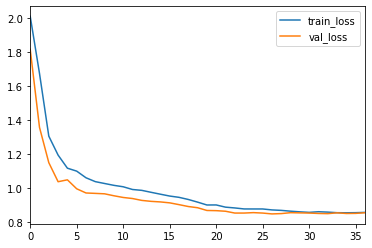In :
_ = res[['train_loss_surv', 'val_loss_surv']].plot()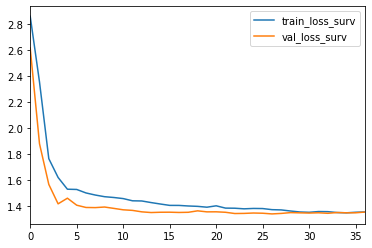In :
_ = res[['train_loss_ae', 'val_loss_ae']].plot()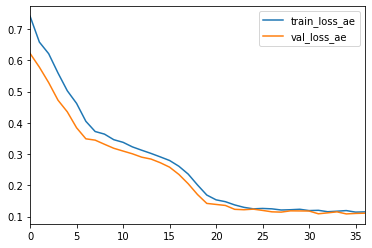## Prediction¶

For prediction, remember that model.predict use the net.predict method, and because we defined it as only the survival part, the predict_surv_df behave as before.

In :
surv = model.interpolate(10).predict_surv_df(x_test)

In :
surv.iloc[:, :5].plot(drawstyle='steps-post')
plt.ylabel('S(t | x)')
_ = plt.xlabel('Time')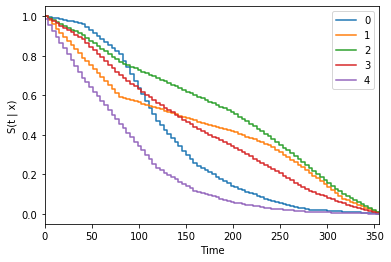Under you can see that the model.predict method gives an array out, as the model.net.predict only gives an array (or tensor).

If we want predictions from the net.forward method, we can use model.predict_net instead.

In :
model.predict(x_test[:2])

Out:
array([[-13.314146  ,  -3.232014  ,  -1.6640139 ,  -0.32413414,
-0.17921695,  -0.24341404,   0.09743847,   0.6742561 ,
-0.08086838,   9.740675  ],
[-12.070942  ,  -1.3327147 ,  -1.0963962 ,  -2.2161934 ,
-2.0299942 ,  -2.0760274 ,  -1.5875834 ,  -0.576796  ,
0.6470416 ,   8.179644  ]], dtype=float32)
In :
model.predict_net(x_test[:2])

Out:
(array([[-13.314146  ,  -3.232014  ,  -1.6640139 ,  -0.32413414,
-0.17921695,  -0.24341404,   0.09743847,   0.6742561 ,
-0.08086838,   9.740675  ],
[-12.070942  ,  -1.3327147 ,  -1.0963962 ,  -2.2161934 ,
-2.0299942 ,  -2.0760274 ,  -1.5875834 ,  -0.576796  ,
0.6470416 ,   8.179644  ]], dtype=float32),
array([[-0.87191707,  1.8376998 ,  0.52234185,  1.4613888 ,  0.8503939 ,
0.93256044,  0.82951564,  0.15673046,  1.0731695 ],
[ 0.05569835, -0.8430163 ,  2.3545551 ,  0.93773973, -1.2269706 ,
0.38499445,  0.79142815,  0.6561861 ,  0.33640665]],
dtype=float32))

We can also pass a function to the predict methods, so we can only get the survival part from predict_net.

In :
model.predict_net(x_test[:2], func=lambda x: x)

Out:
array([[-13.314146  ,  -3.232014  ,  -1.6640139 ,  -0.32413414,
-0.17921695,  -0.24341404,   0.09743847,   0.6742561 ,
-0.08086838,   9.740675  ],
[-12.070942  ,  -1.3327147 ,  -1.0963962 ,  -2.2161934 ,
-2.0299942 ,  -2.0760274 ,  -1.5875834 ,  -0.576796  ,
0.6470416 ,   8.179644  ]], dtype=float32)

## Evaluation (as before)¶

The EvalSurv class contains some useful evaluation criteria for time-to-event prediction. We set censor_surv = 'km' to state that we want to use Kaplan-Meier for estimating the censoring distribution.

In :
ev = EvalSurv(surv, durations_test, events_test, censor_surv='km')


#### Concordance¶

In :
ev.concordance_td('antolini')

Out:
0.6662427402602908

#### Brier Score¶

We can plot the the IPCW Brier score for a given set of times. Here we just use 100 time-points between the min and max duration in the test set. Note that the score becomes unstable for the highest times. It is therefore common to disregard the rightmost part of the graph.

In :
time_grid = np.linspace(durations_test.min(), durations_test.max(), 100)
ev.brier_score(time_grid).plot()
plt.ylabel('Brier score')
_ = plt.xlabel('Time')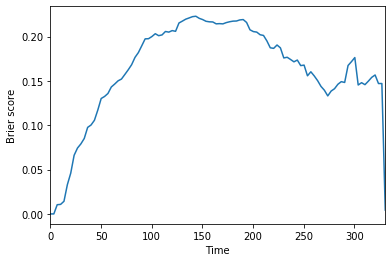#### Negative binomial log-likelihood¶

In a similar manner, we can plot the the IPCW negative binomial log-likelihood.

In :
ev.nbll(time_grid).plot()
plt.ylabel('NBLL')
_ = plt.xlabel('Time')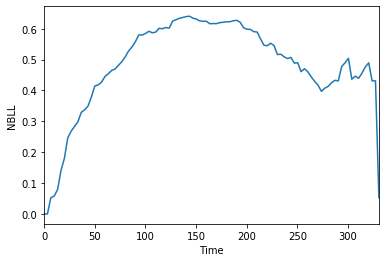#### Integrated scores¶

The two time-dependent scores above can be integrated over time to produce a single score Graf et al. 1999. In practice this is done by numerical integration over a defined time_grid.

In :
ev.integrated_brier_score(time_grid)

Out:
0.16289642991716796
In :
ev.integrated_nbll(time_grid)

Out:
0.4834288744418562

# Next¶

You can now look at other examples of survival methods in the examples folder. Or, alternatively take a look at

In [ ]: